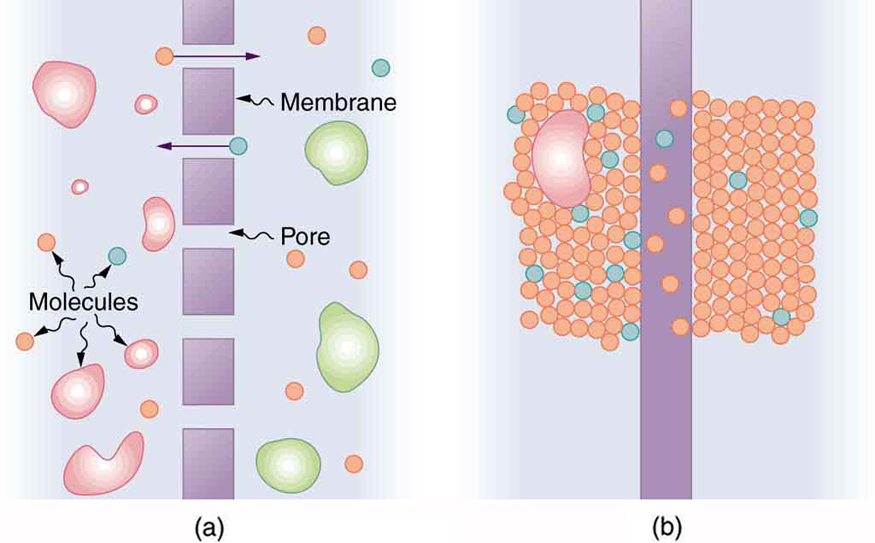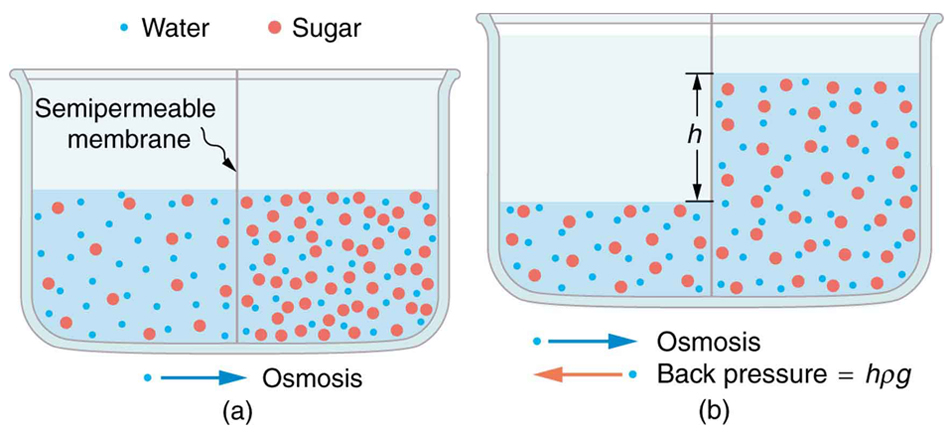# 12.7 Molecular transport phenomena: diffusion, osmosis, and related  (Page 3/12)

 Page 3 / 12(a) A semipermeable membrane with small pores that allow only small molecules to pass through. (b) Certain molecules dissolve in this membrane and diffuse across it.

Osmosis is the transport of water through a semipermeable membrane from a region of high concentration to a region of low concentration. Osmosis is driven by the imbalance in water concentration. For example, water is more concentrated in your body than in Epsom salt. When you soak a swollen ankle in Epsom salt, the water moves out of your body into the lower-concentration region in the salt. Similarly, dialysis    is the transport of any other molecule through a semipermeable membrane due to its concentration difference. Both osmosis and dialysis are used by the kidneys to cleanse the blood.

Osmosis can create a substantial pressure. Consider what happens if osmosis continues for some time, as illustrated in [link] . Water moves by osmosis from the left into the region on the right, where it is less concentrated, causing the solution on the right to rise. This movement will continue until the pressure $\rho \text{gh}$ created by the extra height of fluid on the right is large enough to stop further osmosis. This pressure is called a back pressure . The back pressure $\rho \text{gh}$ that stops osmosis is also called the relative osmotic pressure    if neither solution is pure water, and it is called the osmotic pressure    if one solution is pure water. Osmotic pressure can be large, depending on the size of the concentration difference. For example, if pure water and sea water are separated by a semipermeable membrane that passes no salt, osmotic pressure will be 25.9 atm. This value means that water will diffuse through the membrane until the salt water surface rises 268 m above the pure-water surface! One example of pressure created by osmosis is turgor in plants (many wilt when too dry). Turgor describes the condition of a plant in which the fluid in a cell exerts a pressure against the cell wall. This pressure gives the plant support. Dialysis can similarly cause substantial pressures.(a) Two sugar-water solutions of different concentrations, separated by a semipermeable membrane that passes water but not sugar. Osmosis will be to the right, since water is less concentrated there. (b) The fluid level rises until the back pressure ρ gh size 12{ρ ital "gh"} {} equals the relative osmotic pressure; then, the net transfer of water is zero.

Reverse osmosis and reverse dialysis    (also called filtration) are processes that occur when back pressure is sufficient to reverse the normal direction of substances through membranes. Back pressure can be created naturally as on the right side of [link] . (A piston can also create this pressure.) Reverse osmosis can be used to desalinate water by simply forcing it through a membrane that will not pass salt. Similarly, reverse dialysis can be used to filter out any substance that a given membrane will not pass.

One further example of the movement of substances through membranes deserves mention. We sometimes find that substances pass in the direction opposite to what we expect. Cypress tree roots, for example, extract pure water from salt water, although osmosis would move it in the opposite direction. This is not reverse osmosis, because there is no back pressure to cause it. What is happening is called active transport    , a process in which a living membrane expends energy to move substances across it. Many living membranes move water and other substances by active transport. The kidneys, for example, not only use osmosis and dialysis—they also employ significant active transport to move substances into and out of blood. In fact, it is estimated that at least 25% of the body’s energy is expended on active transport of substances at the cellular level. The study of active transport carries us into the realms of microbiology, biophysics, and biochemistry and it is a fascinating application of the laws of nature to living structures.

## Section summary

• Diffusion is the movement of substances due to random thermal molecular motion.
• The average distance ${x}_{\text{rms}}$ a molecule travels by diffusion in a given amount of time is given by
${x}_{\text{rms}}=\sqrt{2D\text{t}},$

where $D$ is the diffusion constant, representative values of which are found in [link] .

• Osmosis is the transport of water through a semipermeable membrane from a region of high concentration to a region of low concentration.
• Dialysis is the transport of any other molecule through a semipermeable membrane due to its concentration difference.
• Both processes can be reversed by back pressure.
• Active transport is a process in which a living membrane expends energy to move substances across it.

## Conceptual questions

Why would you expect the rate of diffusion to increase with temperature? Can you give an example, such as the fact that you can dissolve sugar more rapidly in hot water?

How are osmosis and dialysis similar? How do they differ?

## Problem exercises

You can smell perfume very shortly after opening the bottle. To show that it is not reaching your nose by diffusion, calculate the average distance a perfume molecule moves in one second in air, given its diffusion constant $D$ to be $1.00×{\text{10}}^{–6}\phantom{\rule{0.25em}{0ex}}{\text{m}}^{2}\text{/s}$ .

$1\text{.}\text{41}×{\text{10}}^{-3}\phantom{\rule{0.25em}{0ex}}\text{m}$

What is the ratio of the average distances that oxygen will diffuse in a given time in air and water? Why is this distance less in water (equivalently, why is $D$ less in water)?

Oxygen reaches the veinless cornea of the eye by diffusing through its tear layer, which is 0.500-mm thick. How long does it take the average oxygen molecule to do this?

$1\text{.}3×{\text{10}}^{2}\phantom{\rule{0.25em}{0ex}}\text{s}$

(a) Find the average time required for an oxygen molecule to diffuse through a 0.200-mm-thick tear layer on the cornea. (b) How much time is required to diffuse $0\text{.500}\phantom{\rule{0.25em}{0ex}}{\text{cm}}^{3}$ of oxygen to the cornea if its surface area is $1\text{.}\text{00}\phantom{\rule{0.25em}{0ex}}{\text{cm}}^{2}$ ?

Suppose hydrogen and oxygen are diffusing through air. A small amount of each is released simultaneously. How much time passes before the hydrogen is 1.00 s ahead of the oxygen? Such differences in arrival times are used as an analytical tool in gas chromatography.

0.391 s

#### Questions & Answers

Calculate the work done by an 85.0-kg man who pushes a crate 4.00 m up along a ramp that makes an angle of 20.0º20.0º with the horizontal. (See [link] .) He exerts a force of 500 N on the crate parallel to the ramp and moves at a constant speed. Be certain to include the work he does on the crate an
Collins Reply
What is thermal heat all about
Abel Reply
why uniform circular motion is called a periodic motion?.
Boniface Reply
when a train start from A & it returns at same station A . what is its acceleration?
Mwdan Reply
what is distance of A to B of the stations and what is the time taken to reach B from A
BELLO
the information provided is not enough
aliyu
Hmmmm maybe the question is logical
yusuf
where are the parameters for calculation
HENRY
there is enough information to calculate an AVERAGE acceleration
Kwok
mistake, there is enough information to calculate an average velocity
Kwok
~\
Abel
what is the unit of momentum
Abel
wha are the types of radioactivity ?
Worku Reply
what are the types of radioactivity
Worku
what is static friction
Golu Reply
It is the opposite of kinetic friction
Mark
static fiction is friction between two surfaces in contact an none of sliding over on another, while Kinetic friction is friction between sliding surfaces in contact.
MINDERIUM
I don't get it,if it's static then there will be no friction.
author
It means that static friction is that friction that most be overcome before a body can move
kingsley
static friction is a force that keeps an object from moving, and it's the opposite of kinetic friction.
author
It is a force a body must overcome in order for the body to move.
Eboh
If a particle accelerator explodes what happens
Eboh
why we see the edge effect in case of the field lines of capacitor?
Arnab
what is wave
Muhammed Reply
what is force
Muhammed
force is something which is responsible for the object to change its position
MINDERIUM
more technically it is the product of mass of an object and Acceleration produced in it
MINDERIUM
wave is disturbance in any medium
iqra
energy is distributed in any medium through particles of medium.
iqra
If a particle accelerator explodes what happens
Eboh Reply
we have to first figure out .... wats a particle accelerator first
Teh
What is surface tension
Subi Reply
The resistive force of surface.
iqra
Who can tutor me on simple harmonic motion
yusuf Reply
on both a string and peldulum?
Anya
spring*
Anya
Yea
yusuf
Do you have a chit-chat contact
yusuf
I dont have social media but i do have an email?
Anya
Which is
yusuf
Where are you chatting from
yusuf
I don't understand the basics of this group
Jimmy
teach him SHM init
Anya
Simple harmonic motion
yusuf
how.an.equipotential.line is two dimension and equipotential surface is three dimension ?
syed Reply
definition of mass of conversion
umezurike Reply
Force equals mass time acceleration. Weight is a force and it can replace force in the equation. The acceleration would be gravity, which is an acceleration. To change from weight to mass divide by gravity (9.8 m/s^2).
Marisa
how many subject is in physics
Adeshina Reply
the write question should be " How many Topics are in O- Level Physics, or other branches of physics.
effiom
how many topic are in physics
Praise
Praise what level are you
yusuf
If u are doing a levels in your first year you do AS topics therefore you do 5 big topic i.e particles radiation, waves and optics, mechanics,materials, electricity. After that you do A level topics like Specific Harmonic motion circular motion astrophysics depends really
Anya
Yeah basics of physics prin8
yusuf
Heat nd Co for a level
yusuf
yh I need someone to explain something im tryna solve . I'll send the question if u down for it
Tamdy Reply
a ripple tank experiment a vibrating plane is used to generate wrinkles in the water .if the distance between two successive point is 3.5cm and the wave travel a distance of 31.5cm find the frequency of the vibration
Tamdy
hallow
Boniface
please send the answer
Boniface
the range of objects and phenomena studied in physics is
Bethel Reply
I don't know please give the answer
Boniface

### Read also:

#### Get the best College physics course in your pocket!

Source:  OpenStax, College physics. OpenStax CNX. Jul 27, 2015 Download for free at http://legacy.cnx.org/content/col11406/1.9
Google Play and the Google Play logo are trademarks of Google Inc.

Notification Switch

Would you like to follow the 'College physics' conversation and receive update notifications?ByByBy